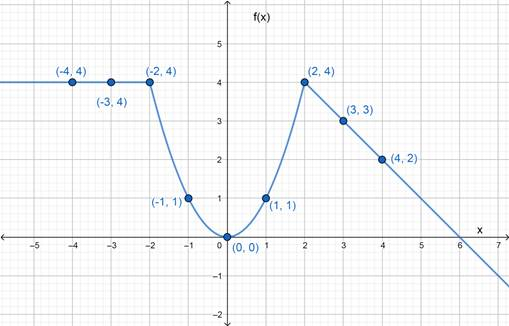# The graph of the given function.### Precalculus: Mathematics for Calcu...

6th Edition
Stewart + 5 others
Publisher: Cengage Learning
ISBN: 9780840068071### Precalculus: Mathematics for Calcu...

6th Edition
Stewart + 5 others
Publisher: Cengage Learning
ISBN: 9780840068071

#### Solutions

Chapter 2.2, Problem 45E
To determine

## To sketch : The graph of the given function.

Expert Solution

### Explanation of Solution

Given information :

f(x)={4             if  x<2x2           if  2x2x+6     if x>2

Graph :

 x −4 −3 −2 −1 0 1 2 3 4 f(x) 4 4 4 1 0 1 4 3 2This is the graph of the given piecewise defined equation.

Interpretation : The part of the graph to the left of x=2 coincides with the graph of f(x)=4 and the part of the graph to the right of x=2 coincides with the graph of f(x)=x+6 . The part of the graph between x=2 and x=2 coincides with f(x)=x2 .

### Have a homework question?

Subscribe to bartleby learn! Ask subject matter experts 30 homework questions each month. Plus, you’ll have access to millions of step-by-step textbook answers!